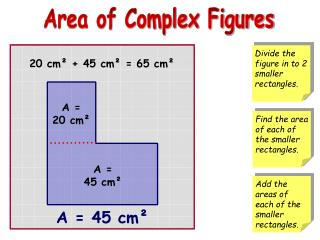DownloadDownload Presentation20 cm²

# 20 cm²

Télécharger la présentation## 20 cm²

- - - - - - - - - - - - - - - - - - - - - - - - - - - E N D - - - - - - - - - - - - - - - - - - - - - - - - - - -
##### Presentation Transcript

1. Divide the figure in to 2 smaller rectangles. Find the area of each of the smaller rectangles. Add the areas of each of the smaller rectangles. Area of Complex Figures 20 cm² + 45 cm² = 65 cm² A = 20 cm² A = 45 cm² A = 45 cm²

2. Divide the figure in to 1 rectangle and 1 square. Find the area of each of the smaller rectangles. Add the areas of each of the smaller rectangles. Area of Complex Figures 44 cm² + 25 cm² = 69 cm² A = 44 cm² A =25 cm² A = 69 cm²

3. Divide the figure in to smaller squares and/ or rectangles. Find the area of each square and/or rectangle. Add the areas of each square and/or rectangle. Area of Complex Figures 9 ft² 9 ft² + + 24 ft² = 42 ft² 3 ft 3 ft × 3 ft 3 ft = 9 ft² 8 ft 8 ft × 3ft 3 ft × 3ft 3 ft = 24 ft² = 9 ft² A = 42 ft²

4. Divide the figure in to smaller squares and/ or rectangles. Find the area of each square and/or rectangle. Add the areas of each square and/or rectangle. Area of Complex Figures 4 yd² + 40 yd² = 44 yd² 2 yd 2 yd × 2 yd 2 yd = 4 yd² 2 yd 6 yd × 15 yd 4 yd = 40 yd² 10 yd A = 44 yd²

5. Divide the figure in to smaller squares and/ or rectangles. Find the area of each square and/or rectangle. Add the areas of each square and/or rectangle. Area of Complex Figures 18 in² 18 in² + + 27 in² = 63 in² 3 in 3 in 3 in × 6 in 3 in × 6 in 6 in = 18 in² = 18 in² 9 in 9 in × 3 in ? 3 in = 27 in² 9 in A = 63 in²

6. Divide the figure in to smaller squares and/ or rectangles. Find the area of each square and/or rectangle. Add the areas of each square and/or rectangle. Area of Complex Figures 16 m² 44 m² + + 9 m² = 69 in² 11 m 3 m × 3 m 3 m 4 m × 4 m = 9 m² 4 m = 16 m² 4 m × 11 m = 44 m² 3 m 4 m 11 in ? 8 m 7 m A = 69 m² 4 m

7. Divide the figure in to smaller squares and/ or rectangles. Find the area of each square and/or rectangle. Add the areas of each square and/or rectangle. Area of Complex Figures 24 ft² 28 ft² + + 15 ft² = 67 ft² 8 ft A = 67 ft² 3 ft × 8 ft 3 ft = 24 ft² 7 ft 4 ft 4 ft × 7 ft = 28 ft² 7 ft 5 ft 5 ft × 3 ft 3 ft ? 4 ft = 15 ft² 9 ft

8. Your Turn! Find the area of the these complex figures by dividing them into smaller squares and/or rectangles. 5 m² + 6 m² = 11 m² 5 m 5 m × 1 m 1 m = 5 m² 1 m 6 m × 1 m 1 m = 6 m² 6 m A = 11 m²

9. Your Turn! Find the area of the these complex figures by dividing them into smaller squares and/or rectangles. 3 in 9 in² 9 in² + + 9 in² = 27 in² 3 in × 3 in 3 in = 9 in² A = 27 in² 3 in 3 in × 3 in 3 in × 3 in 3 in = 9 in² = 9 in²

10. Your Turn! Find the area of the these complex figures by dividing them into smaller squares and/or rectangles. 6 ft 6 ft × 3 ft 3 ft = 18 ft² 3 ft 3 ft × 2 ft 18 ft² 6 ft² + + 18 ft² = 42 ft² 2 ft = 6 ft² A = 42 ft² 6 ft × 3 ft = 18 ft²

11. Your Turn! Find the area of the these complex figures by dividing them into smaller squares and/or rectangles. 16 cm² + 100 cm² = 116 cm² 10 cm A = 116 cm² 10 cm × 10 cm 10 cm = 100 cm² 4 cm 4 cm × 4 cm 4 cm = 16 cm²